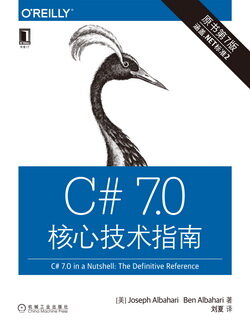# C# 7.0 核心技术指南 (35)：C#语言基础 2.7.3(简化数组初始化表达式)

char[] vowels = {'a','e','i','o','u'}; int[,] rectangularMatrix ={  {0,1,2},  {3,4,5},  {6,7,8}}; int[][] jaggedMatrix ={  new int[] {0,1,2},  new int[] {3,4,5},  new int[] {6,7,8}};

var i = 3;           // i is implicitly of type intvar s = "sausage";   // s is implicitly of type string // Therefore: var rectMatrix = new int[,]    // rectMatrix is implicitly of type int[,]{  {0,1,2},  {3,4,5},  {6,7,8}}; var jaggedMat = new int[][]    // jaggedMat is implicitly of type int[][]{  new int[] {0,1,2},  new int[] {3,4,5},  new int[] {6,7,8}};

var vowels = new[] {'a','e','i','o','u'};   // Compiler infers char[]

var x = new[] {1,10000000000};   // all convertible to long